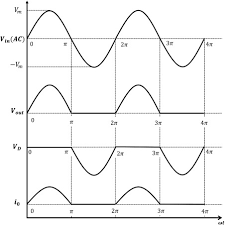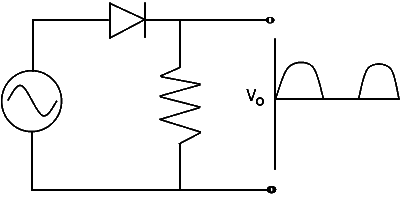### Half Wave Rectifier

In this circuit output voltage can be passed either in negative or positive half cycle. This circuit convert AC signal to DC signal  but efficiency of this circuit is low ie approximate 40 percentParameter of half wave rectifier:

• Output voltage: Average value/dc value/mean value= Area over one period /Total time period

=Vm/𝝅

• Average output current =Vm/𝝅R

• RMS output voltage: It is root mean square value of output voltage

= Vm/2

• RMS output current: It is root mean square value of current

= Vm/2R

• RMS value of AC component Iac(rms) =0.385*Im

• Input power : Pin = Irms*Irms*R

• Efficiency: 𝜼 = Pout/Pin=0.4052

• PIV: IT is maximum voltage across diode in reverse bias condition.

• Output power: Pout =Idc*Idc*R

PIV=Vm

• Ripple factor: 𝜸 = unwanted comp /wanted comp

=  Iac(rms)/Idc

= 1.21